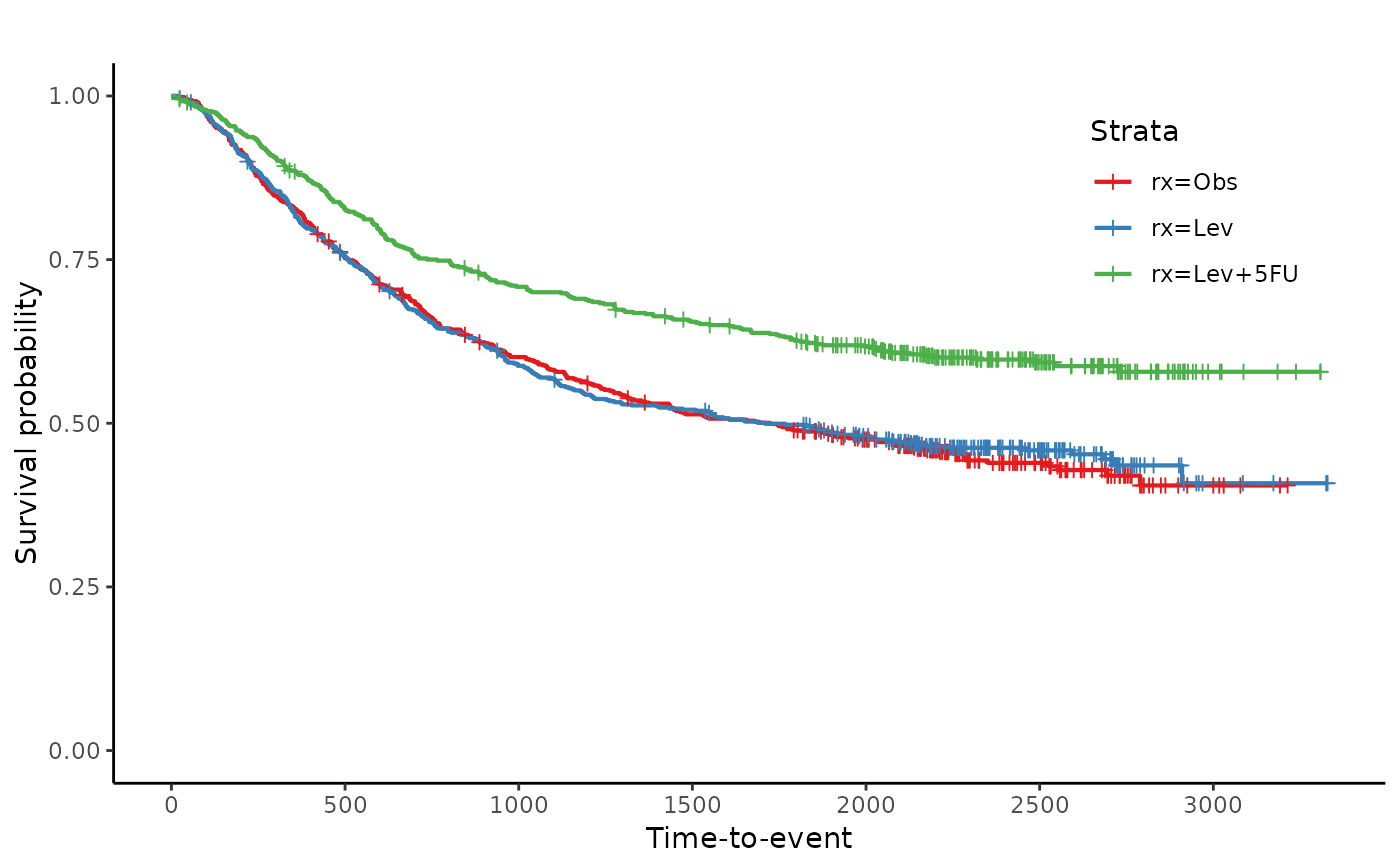Creates a Kaplan-Meier plot with at risk tables below for survfit object.

jskm(
sfit,
table = FALSE,
xlabs = "Time-to-event",
ylabs = "Survival probability",
xlims = c(0, max(sfit$time)), ylims = c(0, 1), surv.scale = c("default", "percent"), ystratalabs = names(sfit$strata),
ystrataname = "Strata",

Plot

DETAILS

Examples

library(survival) data(colon)